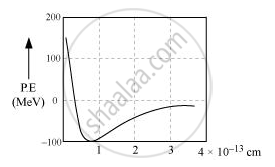Share

# Draw a Plot of Potential Energy of a Pair of Nucleons as a Function of Their Separation.What is the Significance of Negative Potential Energy in the Graph Drawn? - Physics

#### Question

Draw a plot of potential energy of a pair of nucleons as a function of their separation.

what is the significance of negative potential energy in the graph drawn?

#### Solution

The plot of potential energy of a pair of nucleons as a function of their separation is given below:Negative potential energy indicates that the system is bounded.

Is there an error in this question or solution?